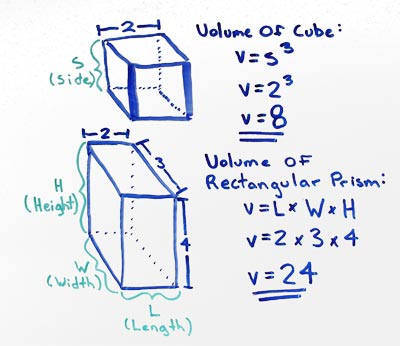# Volume of Cube

## Rectangular Prism Volume

A rectangular prism with a length (L) of 2 a width (W) of 3 and a height (W) of 4 has a volume (V) of 24.

## Rectangular Prism Volume Formula

calculate the volume V of a rectangular prism by multiplying the measured length L by the measured width W and the measured height H

• V = L x W x HThe volume of a rectangular prism can be determined by multiplying Length (L) x Width (W) x Height (H).

### Formulas

What is the formula for the volume of a Cube?

V = S3

What is the formula for the volume of a Rectangular Prism?

V = L x W x H

### Other Formulae

for cubes with side length S

Cube Surface Area

6S2
Cube Face Diagonal
(√2)S
Cube Space Diagonal
(√3)S
S/2
All formulae...